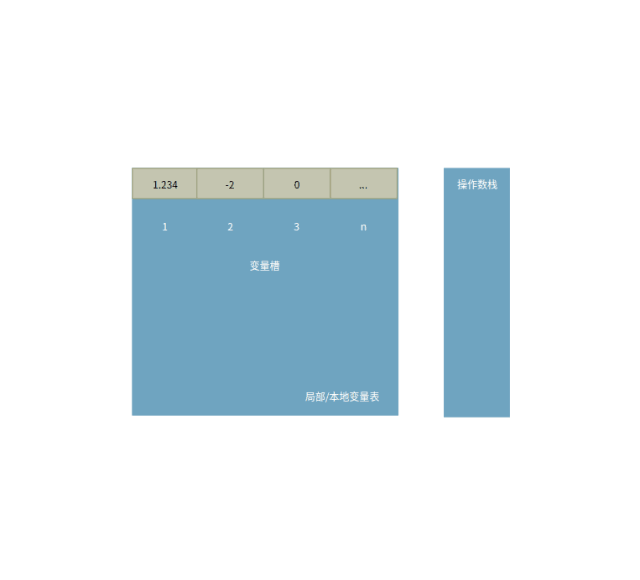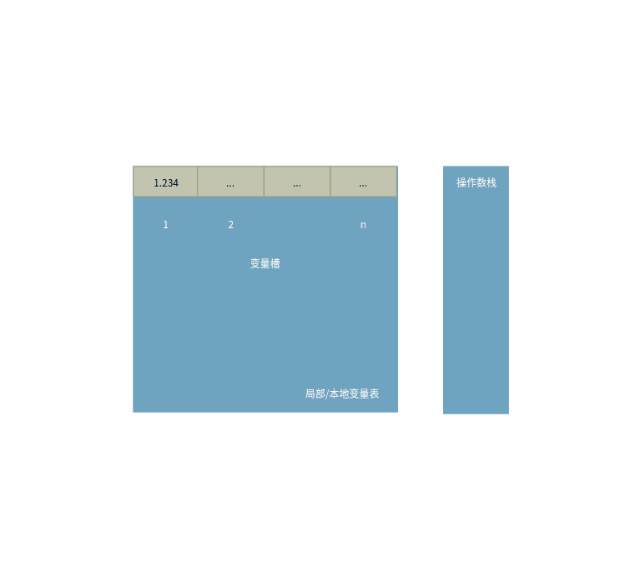• 累计撰写 137 篇文章
• 累计创建 60 个标签
• 累计收到 2 条评论

# 深入理解Java运算符2020-11-05 / 0 评论 / 0 点赞 / 557 阅读 / 3,526 字 / 正在检测是否收录...广告

### 优先级

As the Java programmer's beginning

DescriptionSeparators/OperatorsAssociativity

//短路
if(23 > 24 && 100/0 == 0){
System.out.println("true");
}

//移位
int val = 0x2222;
System.out.println(Integer.toBinaryString(val));
System.out.println(Integer.toBinaryString(~val));

//位
int n = -10;
System.out.println(Integer.toBinaryString(n));
System.out.println(Integer.toBinaryString(n >> 3));
System.out.println(Integer.toBinaryString(n >>> 3));


//Test one
int num = 0;
StringBuilder sb = new StringBuilder();
if (((sb instanceof StringBuilder == num++ > 0) && (++num > 1)) || (num != 2)) {
System.out.println("true");
}
System.out.println("num = " + num);

//Test two
int num = 0;
StringBuilder sb = new StringBuilder();
if (((sb instanceof StringBuilder == num++ > 0) || (++num > 1)) && (num != 2)) {
System.out.println("true");
}
System.out.println("num = " + num);

//Test three
int num = 0;
StringBuilder sb = new StringBuilder();
if (sb instanceof StringBuilder ? false : (false == (((num++ > 0) && (++num > 1)) || (num != 2)))) {
int a = 4;
int b = 3;
int c = a > b ? ++b == b ? a++ : b : a;
System.out.println("a = " + a);
System.out.println("b = " + b);
System.out.println("c = " + c);
}
System.out.println("num = " + num);


Test three同样先在表达式中找出优先级最低的运算符，这里为三目。跟上面以结合性确认先算哪边的方式不同，这里多了一步，需要先算出?左边的真假，才能进一步确认接下来要进入冒号:左边的分支还是右边的分支。

### 基于栈的字节码指令集

float a = 1.234f;
int b = (int) -++a, c = -b + -(int) a++;
b = ++b;
c = c++;
System.out.println(b);
System.out.println(c);


//对应b = ++b
22: iinc          2, 1
26: istore_2

//对应c = c++
28: iinc          3, 1
31: istore_3...
//对应(int) -++a
4: fconst_1
6: dup
7: fstore_1
8: fneg
9: f2i
...
//对应-b + -(int) a++
12: ineg
14: dup
15: fconst_1
17: fstore_1
18: f2i
19: ineg
...### 参考资料

0
1.2.• 0
1.2.